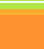back-projection
for CT scanningReconstruction of image by backprojection.
Backprojection applied to a 4 element 2x2 matrix.
Colorado Uni has a useful section on the theory of backprojection and reconstruction.
Texts e.g. wikibooks explain how to construct the matrix of ray-sums from an original matrix.
They then derive the original by
(a) subtracting a background(bg) or offset value from each element
(b) dividing by a number (n) sometimes called the normalisation or renormalisation or rationalisation factor.
Unfortunately, they don't explain how to obtain bg and n.
This will show how to calculate bg and n for a 2x2 matrix.
The same principle applies to any size matrix.
You can test yourself on some 2x2, 3x3 and 4x4 matrices.
 (a) (b) (c) (d) (e) (f) (g) 3 4 7 7 11 4 4 12 3 5 25 28 3 4 1 8 9 9 1 11 4 12 5 8 19 40 1 8
(a) the original matrix
(b) ray sums from the horizontal view placed in the corresponding pixels.
(c), (d), (e) ray sums from views obtained by rotating 45deg clockwise placed in the corresponding pixels.
(f) sum of all the ray sums for each pixel (eg 25=7+11+4+3)
(g) subtract a background(bg) of 16 from pixel and divide each by n=3 to rationalise or normalise it.
How to calculate bg and n
As (f) is the sum of all the CT scans, it is equivalent to the original matrix (a)
Take each pixel of the top row of (f):
we know that if we subtract the background(bg) from each pixel and divide by the normalisation (n), this will give us the magnitudes of the original pixels.
Although we don't know the magnitude of each pixel, we do know their sum (=7)(see matrix(b))
So: (25 - bg)/n + (28 - bg)/n = 7 ie (25+28) - 2bg = 7n
similarly for the 2nd row:
(19 - bg)/n + (40 - bg)/n = 9 ie (19+40) - 2bg = 9n
This gives us 2 equations with 2 unknowns.
Solving gives n=3, bg=16. for this matrix.
In general you can show: n=3 for any 2x2 matrix & bg = sum of all original pixels = 1/7 of sum of all pixels in overall CT scan.

The table shows a matrix of pixel intensities derived from backprojection.
First calculate the background and normalisation factors
Then calculate the original value of each pixel using (Vpix - bg)/n
 (f) CT sum (g) original matrix 3 4 background= 1 8 normalization=

3x3 matrix.
There is no simple solution for a 3x3 matrix. e.g.
 (a) (b) (c) (d) (e) (f) (g) 1 1 1 3 3 3 4 2 1 3 4 3 1 2 4 11 11 11 bg=6 1 1 1 1 2 1 4 4 4 2 4 2 3 4 3 2 4 2 11 16 11 n=5 1 2 1 1 1 1 3 3 3 1 2 4 3 4 3 4 2 1 11 11 11 1 1 1
This can be solved by inspection:
(c) and (e) give the corner pixels as 1, using (b) the centre is 2 and middle of sides = 1. This method cannot be used on larger matrices.
As above: using rows 1 & 2 of (f) and (b):
(11 -bg)/n + (11 -bg)/n + (11 -bg)/n = 3
(11 -bg)/n + (16 -bg)/n + (11 -bg)/n = 4
Hence: n=5 and bg=6

The table shows a matrix of pixel intensities derived from backprojection.
(b) shows the intensities from a horizontal scan
(f) shows the total sum of all 4 scans
Your task is to calculate the original matrix of pixels and complete (g)
First calculate the background and normalisation factors
Then calculate the original value of each pixel using (Vpix - bg)/n
 (b) (f) (g) 11 11 11 n= 4 4 4 11 16 11 bg= 3 3 3 11 11 11

4x4 matrices and larger
Much more complicated and solution depends on lines chosen to correlate with by the above methods.
Therefore, coding is going to be much more complex and may take me some time.
Meanwhile, I've written an Excel spreadsheet which is downloadable to show 2x2, 3x3 and 4x4 matrices, the ray-sums and the results of reducing the raysums by backprojection, including showing the background and normalisation factors.
You can, of course, change the values of the original pixels. Excel then calculates n and bg and shows the result obtained by backprojection.
You can see that many of them suffer from blur.
To be continued... (maybe).